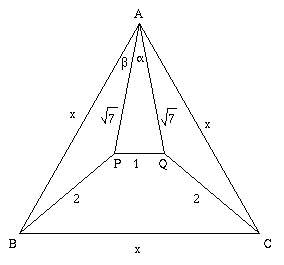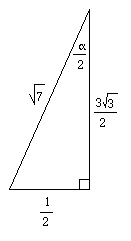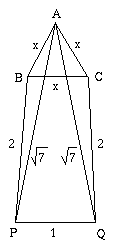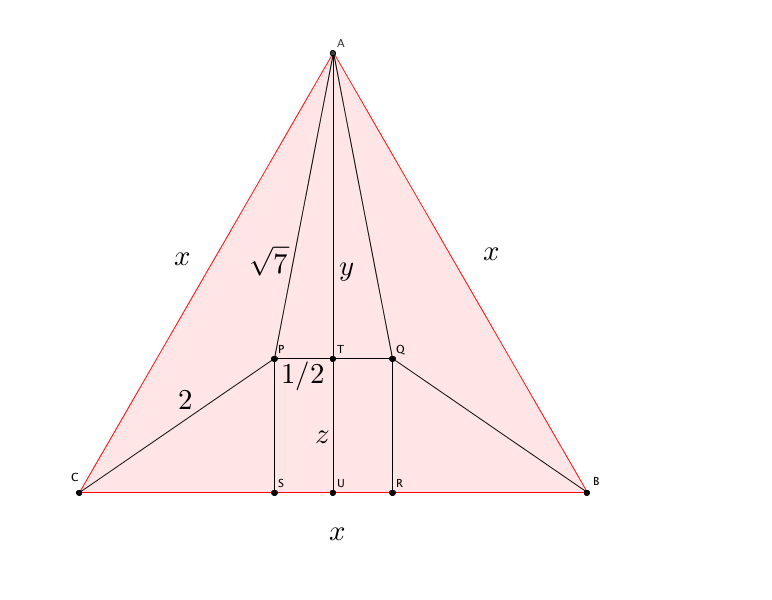#### You may also like### Some(?) of the Parts

A circle touches the lines OA, OB and AB where OA and OB are perpendicular. Show that the diameter of the circle is equal to the perimeter of the triangle### Baby Circle

A small circle fits between two touching circles so that all three circles touch each other and have a common tangent? What is the exact radius of the smallest circle?### Logosquares

Ten squares form regular rings either with adjacent or opposite vertices touching. Calculate the inner and outer radii of the rings that surround the squares.

# Xtra

##### Age 14 to 18Challenge Level

Two solutions to this problem have been forthcoming from different students at the same school - Madras College. Thank you to Mike and Euan who used lots of trigonometry as well as to Thom who likewise resorted to double angles and the cosine rule and reduced the problem to solving a quadratic equation. Thom was also able to show the significance of the two roots.\eqalign{ \beta &=& \frac{\pi}{6} - \frac{\alpha}{2} \\ \cos\beta &=& \frac{\sqrt{3}}{2}\cdot\frac{3\sqrt{3}}{2\sqrt{7}} + \frac{1}{2}\cdot\frac{1}{2\sqrt{7}} \\ \; &=& \frac{10}{4\sqrt{7}} = \frac{5}{2\sqrt{7}}}Using the cosine rule on $\triangle ABP$ \eqalign{ 4 &=& x^2 + 7 - 2x\sqrt{7}\cos\beta \\ \; &=& x^2 + 7 - 5x} Therefore $x^2 - 5x + 3 = 0$ $$x = \frac{5\pm\sqrt{13}}{2}$$ Both solutions satisfy the triangle inequality for $\triangle ABP$, namely $\sqrt{7} - 2 < x < \sqrt{7} + 2$. The diagram can be redrawn to show the trapezium $BPQC$ flipped down producing the much smaller equilateral triangle of side $x$ units.A solution which just needs Pythagoras's Theorem was sent in by Ewan from King Edward VII School, Sheffield. See if you can work it out from the diagram below, then reveal the hidden text to check your answer.The diagram shows the median AU at point A cutting the triangle in half. The length AU is in two parts, $y$ and $z$. Since the triangle is equilateral, $y+z=\frac{\sqrt 3}{2}x$. (You may like to prove this e.g. by trigonometry) To work out $y$ use Pythagoras's Theorem:
\begin{align}
y^2+\left(\frac{1}{2}\right)^2 &=\left(\sqrt 7\right)^2 \\
y^2 &= \frac{27}{4} \\
y &= \frac{3\sqrt 3}{2}
\end{align}
and use $y+z=\frac{\sqrt 3}{2}x$ to give $z = (x-3)\frac{\sqrt 3}{2}$.

Then more Pythagoras's Theorem and our values found above give
\begin{align}
z^2+\left(\frac{x}{2} - \frac{1}{2}\right)^2 &=2^2 \\
\frac{3(x-3)^2}{4} + \frac{(x-1)^2}{4} = 4
\end{align}
which simplifies to get the same equation as Thom and the others: $x^2 -5x + 3 = 0$.

What a simple solution, Well done!